# PHP对文件读取写入的基本操作知识点，另附id传参教学！

## 知识点：

```//打开文件
fopen(string \$filename,string \$mode);
//给文件写入内容
fwrite(\$fp, \$a);
//关闭资源
fclose(\$fp);
//从文件指针中读入一行并解析 CSV 字段
fgetcsv(resource \$handle);```1.创建并打开msg.txt文档

```//a参数为写入方式打开，将文件指针指向文件末尾。如果文件不存在则尝试创建之。
\$fp= fopen('./files/msg.txt','a');```2.前端提交留言：

```<!DOCTYPE html>
<html>
<meta charset="UTF-8">
<title>添加留言</title>
<body>
<form action="write.php" method="post">
标题：
<input type="text" name="title" id=""><br />
内容：
<textarea name="content" id="" cols="30" rows="10"></textarea><br />
<input type="submit" value="提交">
</form>
</body>
</html>```3.将前台提交留言写入msg.txt。

```//拼接写入内容
\$a=\$_POST['title'].','.\$_POST['content']."\n";
//打开文件
\$fp= fopen('./files/msg.txt','a');
//沿着资源写入内容
fwrite(\$fp, \$a);
//关闭资源
fclose(\$fp);
//友好提示
echo 'Is OK';```4.读取文档内容。

```\$fp = fopen('./files/msg.txt', 'r');
echo '<table border="1">';
//fgetcsv函数指针识别到最后没内容会返回false.
while ((\$row = fgetcsv(\$fp)) != false) {
echo '<tr>';
echo '<td>' . \$row . '</td>';
echo '<td>' . \$row . '</td>';
echo '</tr>';
}
echo '</table>';
fclose(\$fp);```5.id传参教学。

```\$fp = fopen('./files/msg.txt', 'r');
echo '<table border="1">';
\$tid = 1;
while ((\$row = fgetcsv(\$fp)) != false) {
echo '<tr>';
echo '<td><a href="'.'read2.php?tid='.\$tid.'">' . \$row . '</td>';
echo '<td>' . \$row . '</td>';
echo '</tr>';
\$tid = \$tid + 1;
}
echo '</table>';```

```\$fp = fopen('./files/msg.txt', 'r');
\$tid = \$_GET['tid'];
\$num = 1;
while ((\$row = fgetcsv(\$fp)) != false) {
if (\$num == \$tid){
echo '<h1>'.\$row.'</h1>';
echo '<p>'.\$row.'</p>';
}
\$num = \$num+1;
}
fclose(\$fp);```## 结束：## 项目源码：

file_demo1.zip大小：2.2 K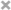### 评论列表

1.访客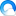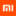【评论达人 LV.6】  @回复

没什么不妥的，稳稳的料！

2.红嘴鸭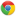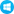【评论达人 LV.1】  @回复

厉害了，学习了， 多谢分享

3.新闻头条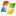【评论达人 LV.1】  @回复

文章不错支持一下吧